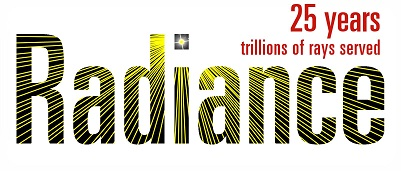# Calculation for CIE Clear Sky and comparing with DIVA for Rhino?

Full content and figures: [Notion – The all-in-one workspace for your notes, tasks, wikis, and databases.]

Hello,

I usually use DIVA for Rhino as a tool for simulating daylighting. To simulate Clear Sky condition with direct sunlight, I choose the option “Clear Sky With Sun (CIE Clear Sky)” in Figure 1.

In academic writing, I take Andrew Marsh’s article “Sky Distribution” as a reference to describe this type of sky luminance distribution. This article describes his calculation methods of his web app “CIE Sky Generator,” including two kinds of calculation methods

My main question is to confirm DIVA for Rhino using which method to calculate “Clear Sky With Sun (CIE Clear Sky).” The details of two calculation methods of Web App “CIE Sky Generator” are as following:

## First calculation method

The first one is to read data in the epw file, the luminance distribution on some day (i.e. Taipei June 21) can be calculated (figure 2). The description on figure 2 said this result is using “direct Beam and diffuse Horizontal” and reverse calculation by “CIE Sky Distribution Equations”.

I am confused that the cell of the “Direct Normal Illuminance” epw file is 5200 lux, but the “Direct Beam” in Web App is 4224 lux (figure 2) . They are different. The cell of “Diffuese Horizontal Illuminance” in epw file is 49200 lux, but the " Diffuse Horrizontal " in Web App is 41548 lux . They are also different.

Is there any reason for this? I guess the reason is as follows:

The Diffuse Horizontal in the Web App is based on the “Diffuse Irradiance” in the epw file. The “Zenith Luminance” is calculated, then the CIE sky distribution formula is used to calculate the entire sky’s luminance distribution.

Finally, integrate the value of Diffuse Horizontal Illuminance. I tried to use Excel to calculate according to its process, but the results look different. IS there any problem in the process or calculation error?

[ Prosess: Diffuse Irradiance → Zenith Luminance → entire luminance distribution → Diffuse Horizontal Illuminance ]

In the first file, I finished calculating luminance distribution for each patch but still don’t know how to integrate them to horizontal illuminance.

Another small question, if I want to add the illuminance value of “Direct Beam” in the Web App to the luminance value of a patch with the sun, should it be directly added like “350.45+75000” (Figure 3)? Or How do I convert?

## Second calculation method

The second one is to use `[Latitude and Longitude and a specific date (such as Taipei June 21)]` and select `[a specific sky type (such as Clear Sky)]` to calculate the luminance distribution result. Here, “Direct Beam” and “Diffuse Horizontal” do not have epw data, so they have default values of 75000 lux and 15000 lux, respectively, as shown in Figure 4.

As I know, the formula of CIE Sky only describes the ratio of zenith luminance to a luminance of any point of the sky (calculation parameters include Standard parameters a to e, sun angle, any point angle).

So the measured zenith luminance (or by Irradiance conversion) is needed to calculate the luminance value of each patch.

So I would like to ask if any journal or document mentioned about these two values of 75000 lux and 15000 lux for “Direct Beam” and “Diffuse Horizontal”, respectively? I would like to ask further, in DIVA for Rhino, if you choose Clear Sky With Sun (CIE Clear Sky), does the luminance distribution calculation also use these two values? Or does it use the first calculation mode (the Irradiance in the epw file)?

There is a document point out that Radiance is based on gendaylit, but I am not sure whether it uses the first or second calculation method, and I am not sure if DIVA for Rhino uses gendaylit foe simulation?

Thank you!